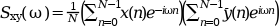Scilab Home page | Wiki | Bug tracker | Forge | Mailing list archives | ATOMS | File exchange
Change language to: English - Português - 日本語 - Русский

# cspect

two sided cross-spectral estimate between 2 discrete time signals using the correlation method

### Syntax

```[sm [,cwp]]=cspect(nlags,npoints,wtype,x [,y] [,wpar])
[sm [,cwp]]=cspect(nlags,npoints,wtype,nx [,ny] [,wpar])```

### Arguments

x

vector, the data of the first signal.

y

vector, the data of the second signal. If `y` is omitted it is supposed to be equal to `x` (auto-correlation). If it is present, it must have the same number of element than `x.`

nx

a scalar : the number of points in the `x` signal. In this case the segments of the x signal are loaded by a user defined function named `getx` (see below).

ny

a scalar : the number of points in the `y` signal. In this case the segments of the `y` signal are loaded by a user defined function named `gety` (see below). If present `ny` must be equal to `nx`.

nlags

number of correlation lags (positive integer)

npoints

number of transform points (positive integer)

wtype

The window type

• `'re'`: rectangular

• `'tr'`: triangular

• `'hm'`: Hamming

• `'hn'`: Hann

• `'kr'`: Kaiser,in this case the wpar argument must be given

• `'ch'`: Chebyshev, in this case the wpar argument must be given

wpar

optional parameters for ```Kaiser and Chebyshev windows:```

• 'kr': ```wpar must be a strictly positive number```

• 'ch': `wpar` must be a 2 element vector `[main_lobe_width,side_lobe_height]with` `0<main_lobe_width<.5`, and `side_lobe_height>0`

sm

The power spectral estimate in the interval `[0,1]` of the normalized frequencies. It is a row array of size `npoints`. The array is real in case of auto-correlation and complex in case of cross-correlation.

cwp

the unspecified Chebyshev window parameter in case of Chebyshev windowing, or an empty matrix.

### Description

Computes the cross-spectrum estimate of two signals `x` and `y` if both are given and the auto-spectral estimate of `x` otherwise. Spectral estimate obtained using the correlation method.

The cross spectrum of two signal x and y is defined to beThe correlation method calculates the spectral estimate as the Fourier transform of a modified estimate of the auto/cross correlation function. This auto/cross correlation modified estimate consist of repeatedly calculating estimates of the autocorrelation function from overlapping sub-segments if the data, and then averaging these estimates to obtain the result.

The number of points of the window is `2*nlags-1.`

For batch processing, the`x` and `y` data may be read segment by segment using the `getx`and `gety` user defined functions. These functions have the following syntax:

`xk=getx(ns,offset)` and `yk=gety(ns,offset)` where `ns` is the segment size and `offset` is the index of the first element of the segment in the full signal.

### Warning

For Scilab version up to 5.0.2 the returned value was the modulus of the current one.

### Reference

Oppenheim, A.V., and R.W. Schafer. Discrete-Time Signal Processing, Upper Saddle River, NJ: Prentice-Hall, 1999

### Examples

```rand('normal');rand('seed',0);
x=rand(1:1024-33+1);
//make low-pass filter with eqfir
nf=33;bedge=[0 .1;.125 .5];des=[1 0];wate=[1 1];
h=eqfir(nf,bedge,des,wate);
//filter white data to obtain colored data
h1=[h 0*ones(1:max(size(x))-1)];
x1=[x 0*ones(1:max(size(h))-1)];
hf=fft(h1,-1);   xf=fft(x1,-1);yf=hf.*xf;y=real(fft(yf,1));
sm=cspect(100,200,'tr',y);
smsize=max(size(sm));fr=(1:smsize)/smsize;
plot(fr,log(sm))```

• pspect — two sided cross-spectral estimate between 2 discrete time signals using the Welch's average periodogram method.
• mese — maximum entropy spectral estimation
• corr — correlation, covariance